# The effect of sputtering RF power on structural, optical and electrical properties of CuO and CuO2 thin films

Document Type: Research Paper

Authors

1 Department of Physics, Faculty of Science, Payame Noor University, Tehran, Iran

2 Department of Physics, Faculty of Science, Kharazmi University, Tehran, Iran

10.22052/JNS.2019.02.005

Abstract

In this paper, the RF power change effect on the structural, optical and electrical properties of CuO thin films prepared by RF reactive magnetron sputtering deposited on glass substrates are studied. At first, the thin films are prepared at 150, 280, 310 and 340W respectively. Then, the films are characterized by XRD, AFM, Uv-visible and four-point probe analysis respectively. The results show that the crystallite size and lattice constant of samples increased from about 20 nm to 59 nm and 4.15 to 4.51 respectively with an increase in RF power from 150 to 340W. The AFM and four-point analysis results show that the samples deposited at 150 and 340W have smooth surfaces and more surface electrical resistance than the samples deposited at 280 and 310W because the dominant phase of samples prepared at 150 and 340W are CuO2 and 280 and 310 are CuO respectively. Also, the results indicate the energy band gap increased from about 2.25 to 2.52eV with an increase in RF power from 150 to 340W and the extinction coefficient of samples prepared at 150W is more than samples prepared at 340W in all of the wavelength.

Keywords

### Full Text

INTRODUCTION

Copper oxide one of the most semiconductors is studied so far. The conductivity of this matter is P-type and the CuO crystal lattice is monoclinic . Most recently, the researchers have produced n-type conductivity of CuO with changing of experimental conditions .

The copper oxide because of its dark color is a photovoltaic matter with high absorption coefficient . CuO is a suitable candidate for solar cell because gap energy of CuO is between 1.2-2.1 eV and CuO has a low electron affinity (3.2 eV) [4, 5]. The researchers in recent researches are trying to produce better solar cells with changing experimental conditions .

In recent decades, the researchers focused on the copper oxide because the copper oxide applications in the industry have increased also trying to determine its properties continues. These researches on the copper oxide are very interesting because electrical, physical and structural properties of CuO, make CuO thin films suitable for many applications such as gas sensor , heterogeneous catalysts , photochromic device , smart windows , Li- ion battery  and LED lamps , catalytic activity  and photosynthesis and Biological activities .

Also, the copper oxide is the best matter to see the Bose-Einstein condensation of excitons . In addition to, the copper oxide is cheaper and more environmentally friendly for industrial application because it’s non-toxic and abundant than another semiconductor such as GaAs and Si Also, it’s more available than SnO2 and In2O3 .

Many processes have been developed for synthesis the CuO Nanostructures, e.g. electrochemical deposition , DC reactive magnetron sputtering , RF reactive magnetron sputtering , vacuum annealing , pulsed laser deposition [20, 21], reactive chemical vapor deposition .

Among these methods the DC reactive magnetron sputtering method is very good technique for prepared thin films, because this method is very controllable and usable in deposition on the large area substrates. In this method physical properties of the samples are control by the deposition parameters such as the Oxygen partial pressure, substrate bias voltage and temperature, sputtering pressure and sputtering power.

These researchers have focused on this material in recent years and investigated structural, optical, electrical and thermal properties [6, 15, 19].

In this paper, we prepared the CuO thin films by RF reactive magnetron sputtering deposited on glass substrates at different RF power. Then, the structural, optical and electrical properties of these layers being studied by XRD, AFM, Uv-visible and four-point probe analysis.

MATERIALS AND METHODS

The CuO thin film is prepared as follow. At first, a pure 99.95% copper target with 101.6 mm diameter and 8 mm thickness is polished using soft sanding and cleaned by aston and ethanol. Then, the argon and oxygen gas is used as work gas sputtering process. Also, the glass substrates cleaned perfectly in aston and etanol solution using an ultrasonic cleaner. The experimental conditions except RF power sputtering are fixed for all of the four steps at during experiment.

The each step of the experiment is 1100 seconds. Any external heat does not apply to the system. Table 1 shows the details of the deposition process.

Then, the structural of samples are investigated using XRD analysis (XRD: Model D8 advance Bruker). Also, the morphology of samples is studied using AFM analysis (AFM: Model DME DS 95-50). The scan zone is 2×2 µm and the figure of AFM is tree dimensional.

In order to study of properties such as extinction coefficient, refractive index, energy gap, transmission spectrum and reflection spectrum are used for spectroscopy analysis. (Uv-visible: Model Gray 500scan). Finally, the surface electrical resistance of samples is studied by four point probe analysis (Four-point-probe: Model 196SYS DMM keithley).

RESULT AND DISCUSSION

Structural properties

XRD analysis

In order to study the structural properties of samples, the samples are considered using XRD at 2θ range equal 10 to 100 degree using Cu Kɑ radiation of wavelength λ=1.5406Å.

Fig. 1 shows that dominant phase of samples is changed of CuO to Cu2O also the peaks of XRD are sharper with increasing RF power sputtering.

Usually the peaks are sharper because of increased grain size and clutter structural of samples [23, 24].

The crystallite size of samples are calculated using Debye-Scherrer equation as fallowing [25, 26]: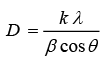(1)

Where λ is the applied X-ray wavelength, θ the diffraction angle in degree and β is the full width at half maximum (FWHM) of the diffraction peak observed in radians. In order to study the lattice constant we used Bragg equation as fallowing :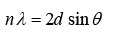(2)

Where n is an integer number, θ the diffraction angle in degree and d is the spacing of lattice planes.

Table 2 shows the result of the crystallite size and lattice constant of samples versus different the RF power sputtering. The results show that the average of the crystallite size of samples increases from 20nm to 59nm also and lattice constant increase from 4.15Å to 4.51Å with increasing of the RF sputtering power respectively.

AFM analysis

The morphology of samples are studied using AFM analysis at scan zone equal 2×2 µm and the figure of AFM is tree dimensional.

Fig. 2 shows the surface roughness of samples. Fig. 2(a-d) shows different factor such as layer roughness, roughness height and surface area of samples are changed with increasing of RF power sputtering.

The results of AFM analysis shown in Table 3. So that, Sa, Sq, Str, Sdq and Sdr is the average watts roughness, the standard deviation (root mean square), the surface isotropy, the gradient of root mean square, and the percentage of surface area respectively. The results indicate that Sdq, Sdr is increased with increasing RF power sputtering. Finally, The AFM results show that the surface of samples with Cu2O as dominant phase (The samples prepared at 150 and 340W) is smoother and also the surface of samples with CuO as dominant phase (The samples prepared at 280 and 310W) is sharper because these samples have more unreactive copper atoms. Therefore, the samples prepared at 280 and 310W exhibit more metallic behavior. Also, the hardness and light transmittance in these layers increase and decrease respectively.

Optical properties

Uv-visible analysis

Transmitting and reflecting spectrum

In order to study the optical properties of the samples such as transmittance rate, reflectance rate, optical energy gap, extinction coefficient, absorption coefficient and the refractive index of the layers the researchers used Uv-visible analysis.

Fig. 3 shows that the prepared samples at 280 and 310 W exhibit more metal since because these samples have more unreactive copper atoms, though produced samples at 150 and 340 W showed better semiconductor behavior. As a result, Fig. 3b shows that transmission spectrum of the layers deposited at 280 and 310 W equal to zero. This is another reason to prove that XRD and AFM sample results which mean the dominant phase of samples are CuO, also these have more hardness and more unreactive copper atoms. Also the results show that increase of power of deposited samples at 150 and 340 W cause a decrease of transmission percent in all wavelengths which is compatible with AFM results. Also, Fig. 3b shows that the transmission percent of the prepared sample at 340 W is less than the prepared sample at 150 W because the former has less surface roughness than later.

In the following, obtained a result from reflection spectrum in Fig. 3a shows that in both sample series which were produced at 280 and 310 W and samples at 150 and 340 W, reflection percent is decreased with the increase of power. This is completely expectable regarding increase size of gradation seen in AFM analysis. In the Table 2, results show that roughness increase with the increase of power from 150 to 310 that will display finer gradation. Only the sample at 340 W does not follow this pattern which is expectable considering the least amount of unreacted copper atom in the sample and the presence of Cu2O phase and maximum semiconductor behavior of the sample. Therefore, the AFM and UV-visible result present acceptable agreement.

Refractive index, extinction coefficient, absorption coefficient and Thickness

In 1983, Swanepol shows that the refractive index of layers with uniform thickness d, refractive index n, and absorption coefficient α and deposition on the substrate with refractive index equal ns can be calculated using maximum and minimum the transmittance spectrum TM(λ), Tm(λ) respectively in the region of weak and medium absorption as follows :

(3)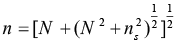where ns is the refractive index of the substrate and also for glass equal 1.50 and N calculated as follows :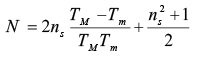(4)

Where Tis a maximum envelope and Tis a minimum envelope. Estimating refraction coefficient of the layer according to two neighboring maximum (or two neighboring minimum), nat point λ1 and n2 at the point λ2, the layer thickness can be obtained by the following relation :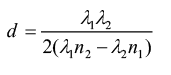(5)

The absorption coefficient can be obtained using thickness and refraction coefficient as fallows: :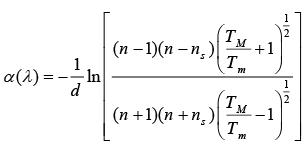(6)

Extinction coefficient also can be obtained using below relationship :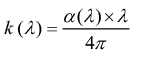(7)

Table 4 shows the results related to thin layers of copper oxide deposited on glass substrate at 150 and 340 W. The results of prepared samples at 280 and 310 W are not considered in the estimation because these layers have more metal properties therefore transmission spectrums of these layers equal to zero.

The results of Table 4 show that the layer thickness, refraction and absorption coefficients of the layers prepared at 150 and 340 W totally increase with increasing power from 150 to 340 W because the dominant phase in these layers is a Cu2O phase and these layers have better semiconductor properties. This result is completely consistence with the obtained conclusion in transmission spectrum which shows that increasing power cause decrease transmission percent and roughness of prepared layer at 340 W is less than that at 150 W in AFM analysis.

Fig. 4 also shows that power increase leads to decrease of extinction coefficient in all wavelengths.

Optical gap energy

With absorption coefficient α(λ) and Taus relationship, it can easily estimate optical energy gap of layers by using below equation :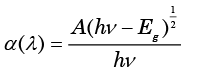(8)

Where, A is a constant value, h is Planck’s constant and Eis energy gap.

Energy gap can be calculated by drawing the tangent line on the curve (αhν)2 versus hv and obtaining intersection with energy axis α=0. As Fig. 5 shows, the optical energy gap of layers increases from 2.19 to 2.31 when power increase from 150 to 340.

Electrical properties

Surface electrical resistance

The surface electrical resistance of deposited samples is measured by the surface resistance measurement device by the four-point method. Measurement results are presented in Table 5. Results obtained from measuring the electrical resistance of deposited copper oxides layers on glass substrate show that deposited layers at 280 and 310 W have less resistance related to deposited layers at 340 and 150 W. Low resistance at 150 and 280 W can be due to the presence of unreacted copper atoms in the layers which are consistence with obtained results of Uv-visible and AFM. Also the results at 150 and 340 W series and 280 and 310 W series show that increase power leads to decrease of surface electrical resistance.

CONCLUSION

In this paper, we studied the RF power change effect on the structural, optical and electrical properties of CuO thin films prepared by RF reactive magnetron sputtering deposited on glass substrates.

The results indicate that the lattice constant, crystallite size and energy band gap of samples increased with an increase in RF power. Also, the results show that extinction coefficient of samples prepared at 150W is more than samples prepared at 340W in all of the wavelength.

The study of structural and electrical properties show that the samples deposited at 150 and 340W have smooth surfaces and more surface electrical resistance than the samples deposited at 280 and 310W because the dominant phase of samples prepared at 150 and 340W are CuO2 and 280 and 310 are CuO respectively.

Finally, we find that deposited samples at 340 and 150 W show better semiconductor behavior but deposited samples at 280 and 310 W display more metal behavior.

CONFLICT OF INTEREST

The authors declare that there are no conflicts of interest regarding the publication of this manuscript.

### References

. Neshkovska R. Electrochromic copper (i) oxide thin film as a candidate for smart window. Acta Technica Corviniensis-Bulletin of Engineering. 2016; 9(2):49.

. Saghatforoush LA, Sanati S, Marandi G, Hasanzadeh M. Synthesis, characterization and catalytic activity of CuO nanostructures using Schiff base copper complexes as a precursor. J Nanostruct. 2013; 3(1):33-41.

. Pansambal S, Deshmukh K, Savale A, Ghotekar S, Pardeshi O, Jain G, Aher Y, Pore D. Phytosynthesis and Biological Activities of Fluorescent CuO Nanoparticles Using Acanthospermum hispidum L. Extract. J Nanostruct. 2017 Jul 1;7(3):165-74.

21. Yousuf AK. Substrate Temperature Effect on the Structure, Morphological and Optical Properties of CuO/Sapphire Thin Films Prepared by Pulsed Laser deposition. Ibn AL-Haitham Journal For Pure and Applied Science. 2017; 27(2):78-86.

25. Razeghizadeh A, Mahmoudi Ghalvandi M, Sohillian F, Rafee V. The Effect of Substrate on Structural and Electrical Properties of Cu3N Thin Film by DC Reactive Magnetron Sputtering. Phys Chem Res. 2017; 5(3):497-504.

26. Rafee, v.; razeghizadeh, a.; Kazeminezhad, i.; Zalaghi, L., Growth and Optical Properties Investigation of Pure and Al-doped SnO2 Nanostructures by Sol-Gel Method. IRAN J CHEM CHEM ENG, 2017.

29. Burns, G. “Solid State Physics Academic Press Inc.” New York (1985).